/  注册

# mysql中聚集索引、辅助索引、覆盖索引、联合索引怎么用

### 聚集索引（Clustered Index）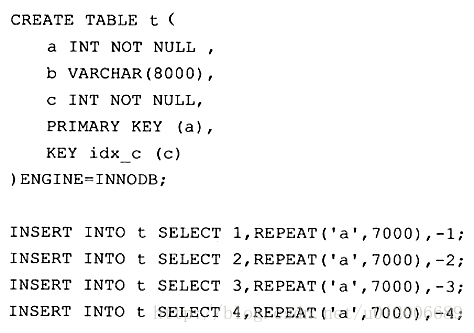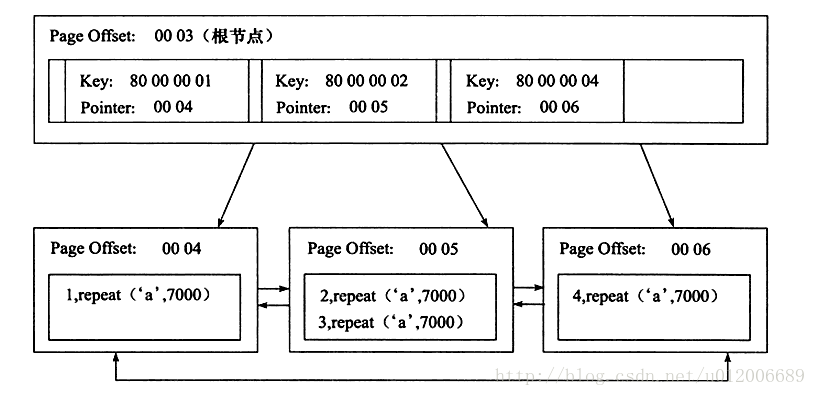The leaf nodes of a clustered index are referred to as data pages, each of which is linked by a doubly linked list, and the data pages are arranged in the order of primary keys.。

### 辅助索引(Secondary Index)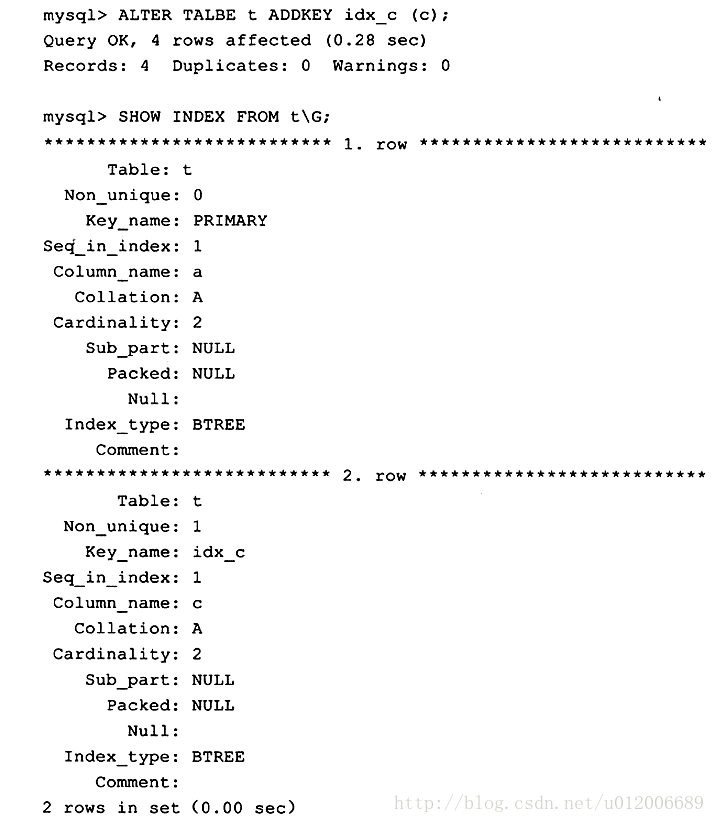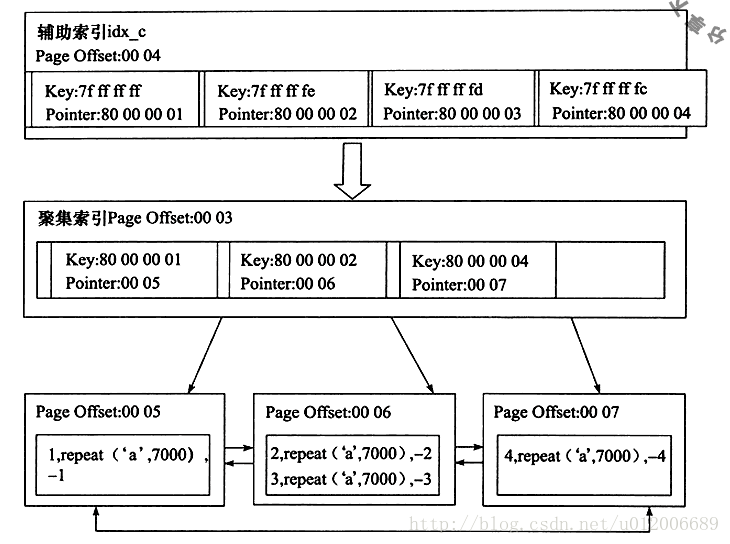### 覆盖索引（Covering index）

• 可以减少大量的IO操作

`select a from where c = -2;`

• 有助于统计

```  CREATE TABLE `student` (
`id` bigint(20) NOT NULL,
`name` varchar(255) NOT NULL,
`age` varchar(255) NOT NULL,
`school` varchar(255) NOT NULL,
PRIMARY KEY (`id`),
KEY `idx_name` (`name`),
KEY `idx_school_age` (`school`,`age`)
) ENGINE=InnoDB DEFAULT CHARSET=utf8;```

`select count(*) from student`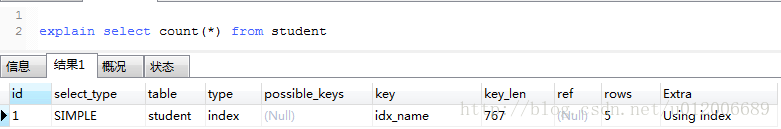key和Extra显示使用了idx_name这个辅助索引。

`select * from student where age > 10 and age < 15`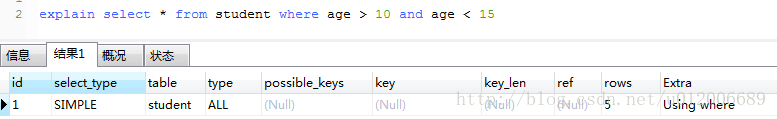`select count(*) from student where age > 10 and age < 15`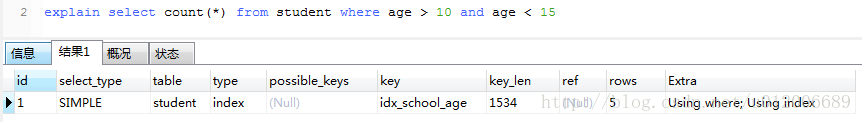### 联合索引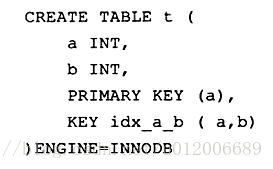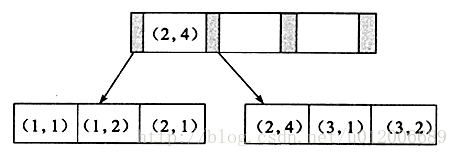```select * from table where a=xxx and b=xxx ;

select * from table where a=xxx;```

`select * from table where b=xxx`

```create table buy_log(
userid int not null,
)ENGINE=InnoDB;

`select * from buy_log where user_id = 2；`

`select * from buy_log where user_id = 2 order by buy_date desc;`

`select * from buy_log where user_id = 2 order by buy_date desc;`

• 相关标签：MySQL
• 相关文章

相关视频